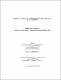## On the determination of sparse Hessian matrices using multi-coloring2016
##### Authors
Mithila, Nasrin Hakim
University of Lethbridge. Faculty of Arts and Science
##### Publisher
Lethbridge, Alta : University of Lethbridge, Dept. of Mathematics and Computer Science
##### Abstract
Efficient determination of large sparse Hessian matrices leads to solving many optimization problems. Exploiting sparsity and symmetry of the Hessian matrix can reduce the number of function evaluations required to determine the matrix. This sparse matrix determination problem can be posed as a graph coloring problem. Graph formulation of the problem using an appropriate model can lead to a better exposition of the matrix compression heuristics.
##### Keywords
direct determination method , multi-coloring , sparse matrix , symmetric Hessian matrices , symmetry-exploiting